# Weka

Weka is an open-source project in machine learning, Data Mining.

Weka is a comprehensive collection of machine-learning algorithms for data mining tasks written in Java.

The algorithms can either be applied directly to a dataset or called from your own Java code.

Weka contains tools for data pre-processing, classification, regression, clustering, association rules, and visualization.

## Weka

### Classifier

#### Output

The more_options menu on the Classify panel can be used to customized the output. Depending on the setup, Weka will generate one or more of the following sections:

• Run information (is always present)
• Stratified cross-validation. The “Folds” parameter is used for stratified cross-validation.
• Evaluation on test set (is present when a separate test set is used)
• Evaluation on training set
• Summary
• Classifier model (full training set)
• Predictions on test data
• Detailed accuracy by class
• Source code

When using cross-validation, Weka prints a model built on the full dataset. The statistics, however, are calculated from the various train/test splits. This can be confusing, because the model stay the same regardless of the number of folds or the value of the random seed.

#### More options

The more_options menu on the Classify panel gives the following options:

• 1 Output model. The “Output model” option toggles whether or not the model built on the full dataset is printed.
• 2 Output per-class stats
• 3 Output entropy evaluation measures
• 5 Store predictions for visualization: This option makes Weka save the classifier's predictions on the test data, and if the model is a tree it saves them at the appropriate leaves. With a very large test set, you might want to turn this off. If you plan to visualize the decision tree produced by J48, this option should you enable to see the classifier's errors on the tree
• 6 Output predictions: This prints a table with actual and predicted values for each instance of the test data. should be set in order to see how the learned classifier deals with each instance in a supplied test set.
• 7 Output additional attributes option should you configure if you want the classifier's predictions to show values of attributes other than the class. Here you can specify a comma-separated range of attribute indices whose values will be included along with the actual and predicted class values. For example, the specification “first-3,10,12-14” would include attributes 1, 2, 3, 10, 12, 13, 14.
• 8 Cost-sensitive evaluation
• 9 Random seed for XCal / % Split
• 10 Preserve order for % Split
• 11 Output source code: will generate Java code that represents the model produced by the classifier. This section prints Java code for the model built on the full training set. This code can be embedded in other Java applications.

## Documentation / Reference

Discover More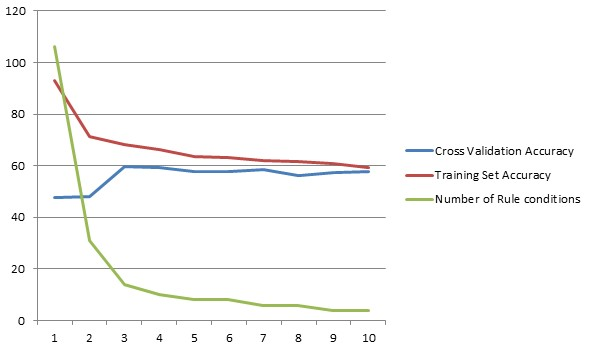Machine Learning - (One|Simple) Rule - (One Level Decision Tree)

One Rule is an simple method based on a 1‐level decision tree described in 1993 by Rob Holte, Alberta, Canada. really simple so small/noisy/complex that nothing can be learned from them ...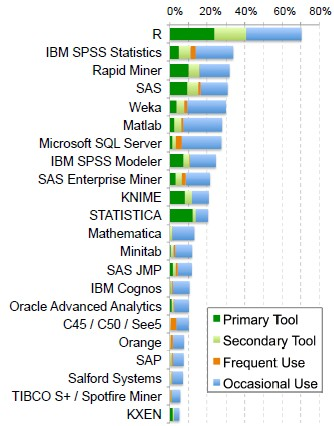Machine Learning - Data Mining (Software, Library and Framework)

This sections contains software library or framework that contains the implementation of machine learning algorithm. See Data...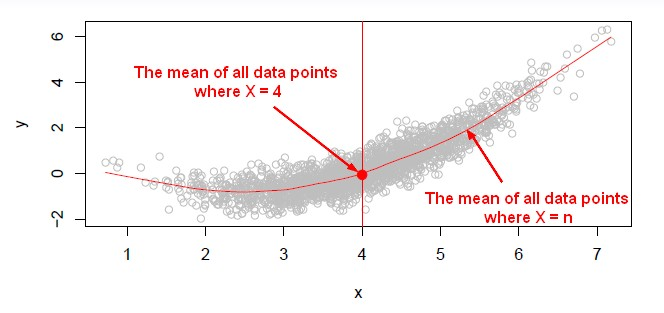Machine Learning - K-Nearest Neighbors (KNN) algorithm - Instance based learning

“Nearest‐neighbor” learning is also known as “Instance‐based” learning. K-Nearest Neighbors, or KNN, is a family of simple: classification and regression algorithms based on Similarity...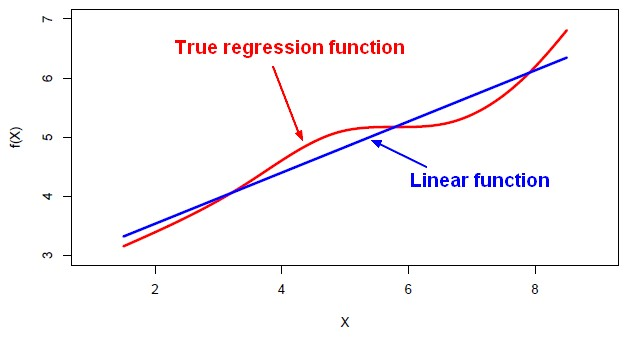Machine Learning - Linear (Regression|Model)

Linear regression is a regression method (ie mathematical technique for predicting numeric outcome) based on the resolution of linear equation. This is a classical statistical method dating back more...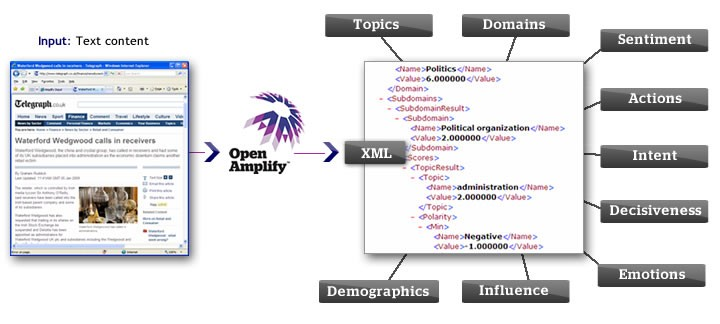NLP - (Software| API )

Apache Nutch: open source web crawler (Nutch can crawl and post to Apache Solr for search/index.) Apache Tika: detects and extracts metadata and text from over a thousand different file types (such as...